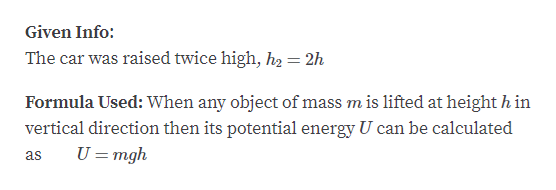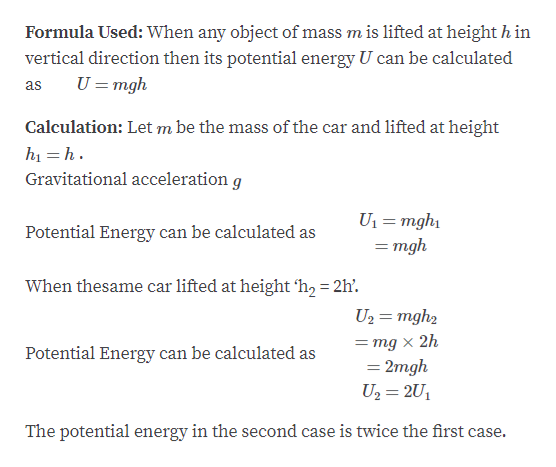# A car is raised a certain distance in a service-station lift and therefore has potential energy relative to floor. If it were raised twice as high, how much potential energy would it have relative to floor ?

Question
11 views

A car is raised a certain distance in a service-station lift and therefore has potential energy relative to floor. If it were raised twice as high, how much potential energy would it have relative to floor ?

check_circle

Step 1help_outlineImage TranscriptioncloseGiven Info: The car was raised twice high, h2 = 2h Formula Used: When any object of mass m is lifted at height h in vertical direction then its potential energy U can be calculated U =mgh as fullscreen
Step 2help_outlineImage TranscriptioncloseFormula Used: When any object of mass m is lifted at height h in vertical direction then its potential energy U can be calculated U = mgh as Calculation: Let m be the mass of the car and lifted at height hị = h . Gravitational acceleration g U1 = mgh1 = mgh otential Energy can be calculated as When thesame car lifted at height 'h, = 2h'. U2 = mgh2 = mg x 2h = 2mgh U2 = 2U1 Potential Energy can be calculated as The potential energy in the second case is twice the first case. fullscreen

### Want to see the full answer?

See Solution

#### Want to see this answer and more?

Solutions are written by subject experts who are available 24/7. Questions are typically answered within 1 hour.*

See Solution
*Response times may vary by subject and question.
Tagged in

### Work,Power and Energy